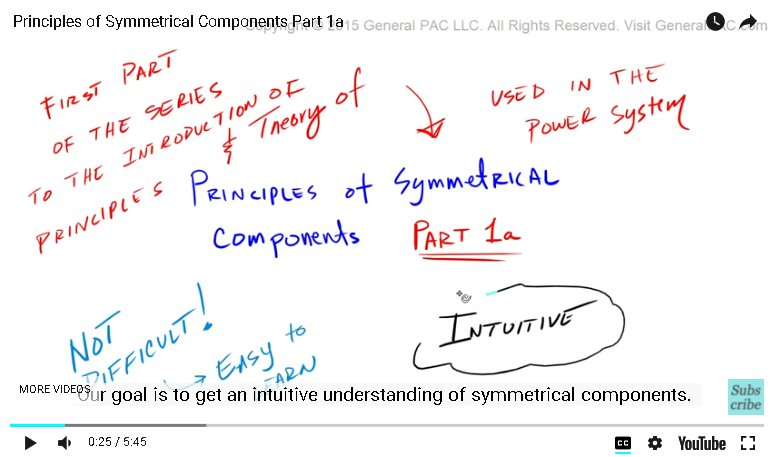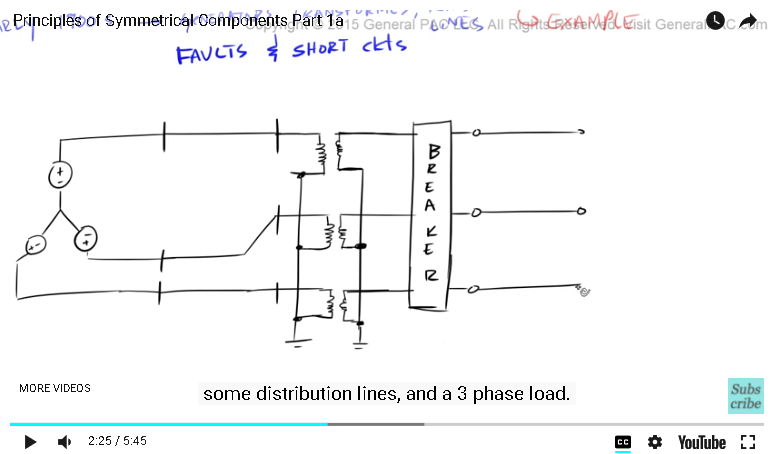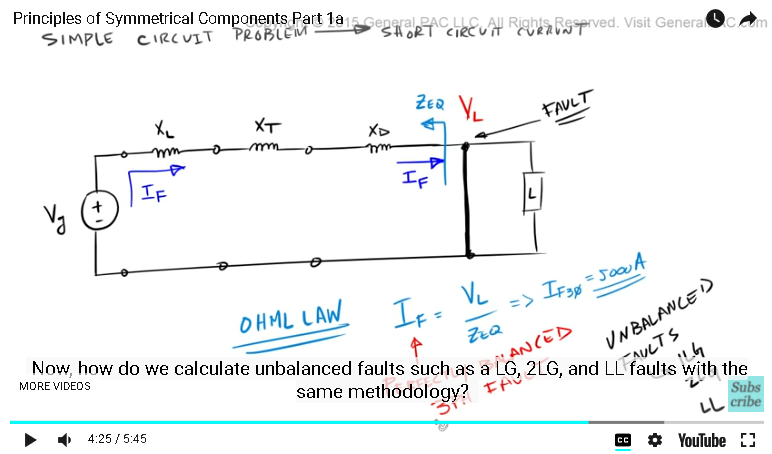### Resources Section

This video tutorial does not items in the resources section

Do you have a question? Click on the "Questions & Answers forum" and ask away!

This video was brought to you by GeneralPAC.com, making power systems Intuitive, Open and Free for Everyone, Everywhere. Consider subscribing and supporting through patreon.com/GeneralPAC. This is a mechanism for you to support us financially so we can continue making high quality power system video tutorials. Our corporate sponsor for this topic is AllumiaX.com from Seattle, Washington. Contact them for industrial and commercial power system studies.

Principles of symmetrical components – Part 1a.

This is the first part of the series, the introduction of principles and theory of symmetrical components used in the power system.

Now, understanding symmetrical components is not difficult. Is actually quite easy to learn, but it sometimes presented in a most confusing and impractical manner. Our goal is to get intuitive understanding of symmetrical components.In this introductory series, we will go step by step and explain what symmetrical components are? the value of symmetrical components, how do we use them in setting circuit breakers and relays? We will look at some of the examples of symmetrical components.

Remember our principle goal is to get intuitive understanding. Now, If you find this video tutorial useful, please subscribe to the GeneralPac channel by clicking on the subscribe button on the bottom right corner of the screen.

In this part, let’s try to answer our first fundamental question, what are symmetrical components? the short answer to that question is the symmetrical components simply transform the unbalanced set of phasors into a balanced set of symmetrical components, which are called as positive sequence components, negative sequence components and zero sequence components.

Now this is the first time that you have been exposed the symmetrical components. This explanation means nothing to you and that’s just the nature of learning something new.

So let’s get a better picture of this definition by asking another question, why are symmetrical components so valuable? and the best way to understand the value of symmetrical components which is a language of some power system engineers.

Let’s give an examples without symmetrical components available to us.

In the early 1900’s before the discovery of symmetrical components, we still had generators, transformers, transmission lines and most importantly faults and short circuits.

Let’s start this example by drawing a simple three-line diagram, we will draw a three phase generators, the generators are connected to a short transmission line, then we have a step-down power transformer connected Y grounded, Y-grounded.

We have an AC three phase circuit breaker on the secondary side of the transformer, some distribution line dedicated to the three phase load.As a power systems engineer, we are asked to set the circuit breaker, so it trips for all types of faults on the distribution line feeding our load.

We are giving a system parameter, like line impedance for example, now the question comes up, right, what are all types of faults mean?

In a power system, there can exist a line to ground fault, a two line to ground faults, a line to line fault and lastly a three-phase fault. Let’s explain this in more detail later.

So we are arrogantly tell that yep, this can be done. We can easily calculate the magnitudes of all of the fault types with no problem.

Remember, this is the early 1900’s before symmetrical components were discovered. To determine the fault current, we begin by drawing the equivalent single phase diagram.

Right, in like a simple phase circuit problem, we expect to calculate the short circuit current, so we draw our voltage source which represents our generator impedances of the transmission line, impedance of the transformer, impedance of the distribution line and the impedance of our load.

Now, if we had a fault at the terminal of our load, which means that we have a short circuit here, we should expect the huge short circuit current that comes from our generator and feeds our fault, to calculate this current, we take the voltage at the terminal of our load and divide by the equivalent impedance seen at the point of the fault.

Right, this is the ohm’s law, plain and simple. Current equals voltage over impedance and that makes total sense and suppose, we get 5000 Amps of three-phase fault current.

Wow, that was pretty easy, but my friends we have simply calculated a perfectly balanced 3 phase fault. Now how do we calculate the unbalanced fault, such as a line to ground, a two line to ground sand line to line fault with a same methodology. That is the point. That is the defining point.Without symmetrical components, we can only calculate balanced three phase fault types. Without symmetrical components, it will be extremely difficult for us to even attempt to calculate unbalanced faults like the line to ground faults, a two line to ground faults and a line to line fault types.

So in this example, what is the value of symmetrical components, with it we can easily calculate both balanced and unbalanced faults and we are able to properly set the breakers for our all expected fault types to distribution lines and that my friends is an extremely critical for the reliability, security and the integrity of the power system.

But that was just a one brief conceptual introduction to symmetrical components. In this series, we will go step by step and explain how it all works in the most intuitive manner possible.

In the next part, part 1b, we will take a step back and describe the balanced set of phasors and review basic operations. This video was brought to you by GeneralPac.co, making power systems protection, automation and controls intuitive.

#### Greetings from the GeneralPAC Team!

We make high-quality Power Systems Video Tutorials on complex topics that are free and open to everyone!  Thank you so much for supporting us through Patreon so we can continue doing good and valuable work.

What is Patreon and why do we use it?

Patreon is a fantastic portal that allows our fans and community to make monthly contribution (like Netflix subscription) so we can continue creating high-quality power systems video tutorials. In return, you get access to incredible perks like voting on future topics, getting your questions answered, access to VIP Q/A webinars with the creators of GeneralPAC, and much more! We THANK YOU for supporting us

Why do we need your support?

An incredible amount of time and effort is needed to develop high-quality video tutorials. Each video (Part 1 for example) takes approximately 10 hours to complete which includes learning the concept ourselves, brainstorming creative ways to teach and explain the concepts, writing the script, audio recording, video recording, and editing. It's no wonder why Hundreds-of-Thousands of people have watched, liked, subscribed, and left positive comments on Youtube channel. Your support truly makes all the difference.